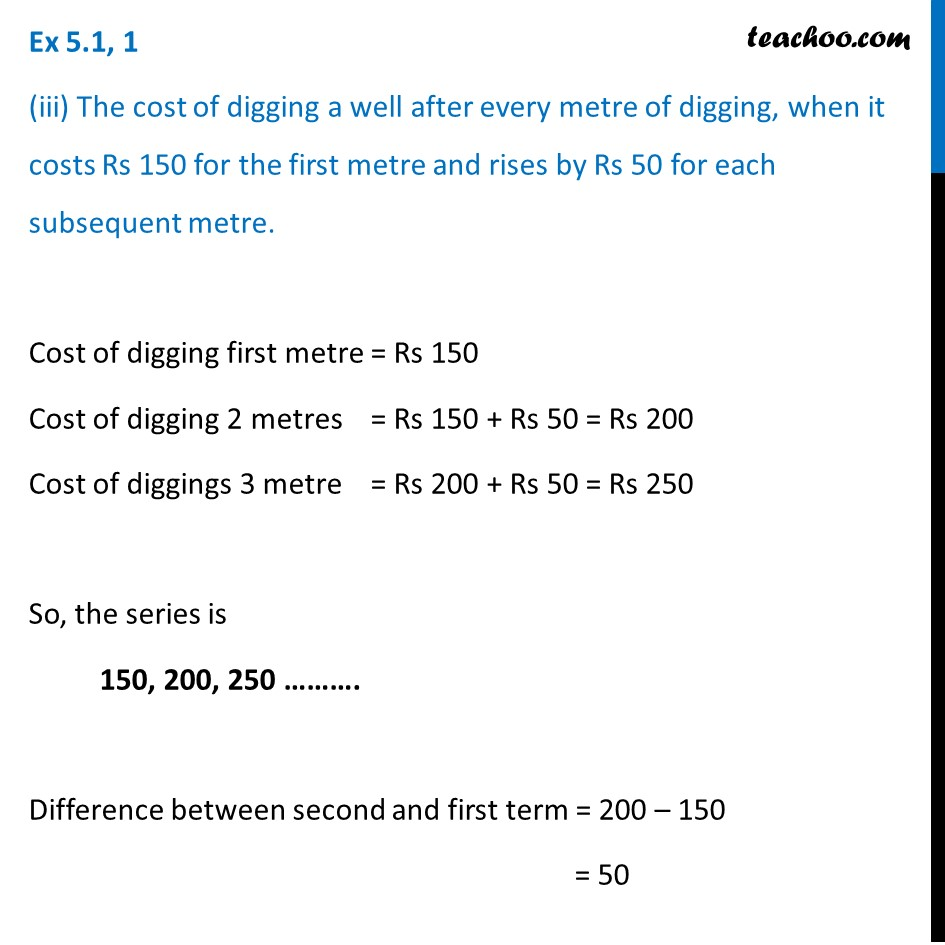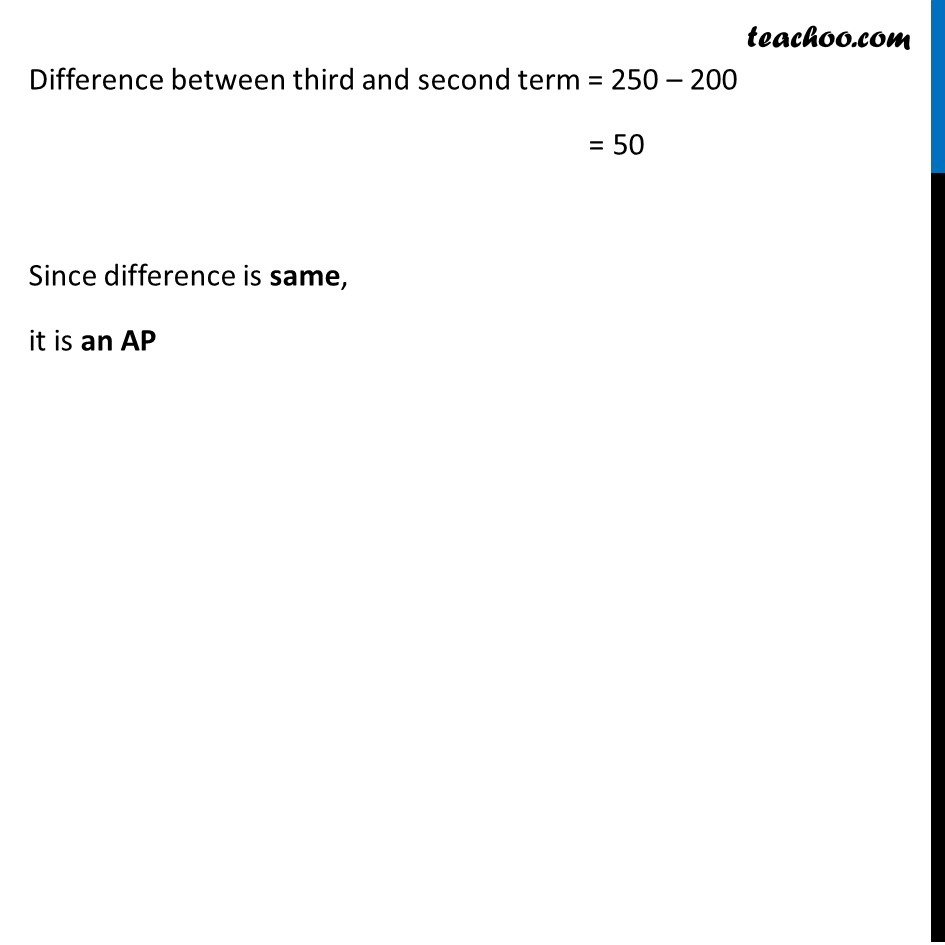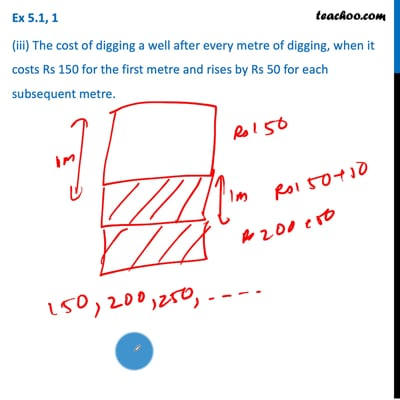Ex 5.1

Chapter 5 Class 10 Arithmetic Progressions
Serial order wiseThis video is only available for Teachoo black users

Introducing your new favourite teacher - Teachoo Black, at only ₹83 per month

### Transcript

Ex 5.1, 1 (iii) The cost of digging a well after every metre of digging, when it costs Rs 150 for the first metre and rises by Rs 50 for each subsequent metre. Cost of digging first metre = Rs 150 Cost of digging 2 metres = Rs 150 + Rs 50 = Rs 200 Cost of diggings 3 metre = Rs 200 + Rs 50 = Rs 250 So, the series is 150, 200, 250 ………. Difference between second and first term = 200 – 150 = 50 Difference between third and second term = 250 – 200 = 50 Since difference is same, it is an AP